Paul's Online Notes
Home / Calculus I / Applications of Integrals / Volumes of Solids of Revolution / Method of Rings
Show Mobile Notice Show All Notes Hide All Notes
Mobile Notice
You appear to be on a device with a "narrow" screen width (i.e. you are probably on a mobile phone). Due to the nature of the mathematics on this site it is best views in landscape mode. If your device is not in landscape mode many of the equations will run off the side of your device (should be able to scroll to see them) and some of the menu items will be cut off due to the narrow screen width.

### Section 6.3 : Volume With Rings

6. Use the method of disks/rings to determine the volume of the solid obtained by rotating the region bounded by $$y = 10 - 6x + {x^2}$$, $$y = - 10 + 6x - {x^2}$$, $$x = 1$$and $$x = 5$$ about the line $$y = 8$$.

Show All Steps Hide All Steps

Start Solution

We need to start the problem somewhere so let’s start “simple”.

Knowing what the bounded region looks like will definitely help for most of these types of problems since we need to know how all the curves relate to each other when we go to set up the area formula and we’ll need limits for the integral which the graph will often help with.

Here is a sketch of the bounded region with the axis of rotation shown.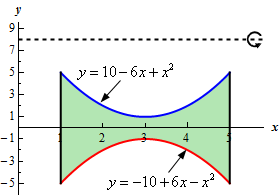Hint : Give a good attempt at sketching what the solid of revolution looks like and sketch in a representative ring.

Note that this can be a difficult thing to do especially if you aren’t a very visual person. However, having a representative ring can be of great help when we go to write down the area formula. Also, getting the representative ring can be difficult without a sketch of the solid of revolution. So, do the best you can at getting these sketches.

Show Step 2

Here is a sketch of the solid of revolution.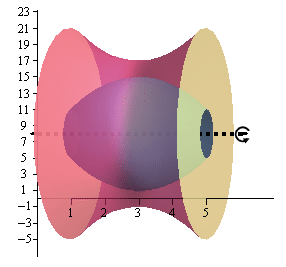Here are a couple of sketches of a representative ring. The image on the left shows a representative ring with the front half of the solid cut away and the image on the right shows a representative ring with a “wire frame” of the back half of the solid (i.e. the curves representing the edges of the of the back half of the solid).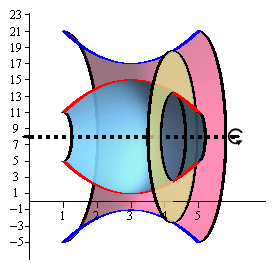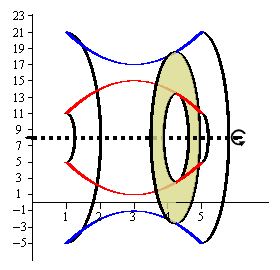Hint : Determine a formula for the area of the ring.
Show Step 3

We now need to find a formula for the area of the ring. Because we are using rings that are centered on a horizontal axis (i.e. parallel to the $$x$$-axis) we know that the area formula will need to be in terms of $$x$$. Therefore, the equations of the curves will need to be in terms of $$x$$ (which in this case they already are).

Here is another sketch of a representative ring with all of the various quantities we need put into it.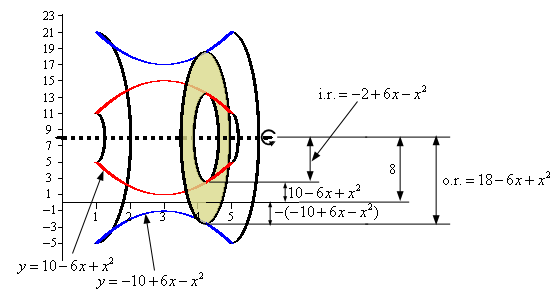From the sketch we can see the ring is centered on the line $$y = 8$$ and placed at some $$x$$.

The inner radius of the ring is then the distance from the axis of rotation to the curve defining the inner edge of the solid. To determine a formula for this first notice that the axis of rotation is a distance of 8 from the $$x$$-axis. Next, the curve defining the inner edge of the solid is a distance of $$y = 10 - 6x + {x^2}$$ from the $$x$$-axis. The inner radius is then the difference between these two distances or,

${\mbox{Inner Radius}} = 8 - \left( {10 - 6x + {x^2}} \right) = - 2 + 6x - {x^2}$

The outer radius is computed in a similar manner. It is the distance from the axis of rotation to the $$x$$-axis (a distance of 8) and then it continues below the $$x$$-axis until it reaches the curve defining the outer edge of the solid. So, we need to add these two distances but we need to be careful because the “lower” function is in fact negative value and so the distance of the point on the lower function from the $$x$$-axis is in fact : $$- \left( { - 10 + 6x - {x^2}} \right)$$ as is shown on the sketch. The negative in front of the equation makes sure that the negative value of the function is turned into a positive quantity (which we need for our distance). The outer radius is then the sum of these two distances or,

${\mbox{Outer Radius}} = 8 - \left( { - 10 + 6x - {x^2}} \right) = 18 - 6x + {x^2}$

The area of the ring is then,

\begin{align*}A\left( x \right) & = \pi \left[ {{{\left( {{\mbox{Outer Radius}}} \right)}^2} - {{\left( {{\mbox{Inner Radius}}} \right)}^2}} \right]\\ & = \pi \left[ {{{\left( {18 - 6x + {x^2}} \right)}^2} - {{\left( { - 2 + 6x - {x^2}} \right)}^2}} \right] = \pi \left( {320 - 192x + 32{x^2}} \right)\end{align*} Show Step 4

The final step is to then set up the integral for the volume and evaluate it.

From the graph from Step 1 we can see that the “first” ring in the solid would occur at $$x = 1$$ and the “last” ring would occur at $$x = 5$$. Our limits are then : $$1 \le x \le 5$$.

The volume is then,

$V = \int_{1}^{5}{{\pi \left( {320 - 192x + 32{x^2}} \right)\,dx}} = \left. {\pi \left( {320x - 96{x^2} + \frac{{32}}{3}{x^3}} \right)} \right|_1^5 = \require{bbox} \bbox[2pt,border:1px solid black]{{\frac{{896}}{3}\pi }}$